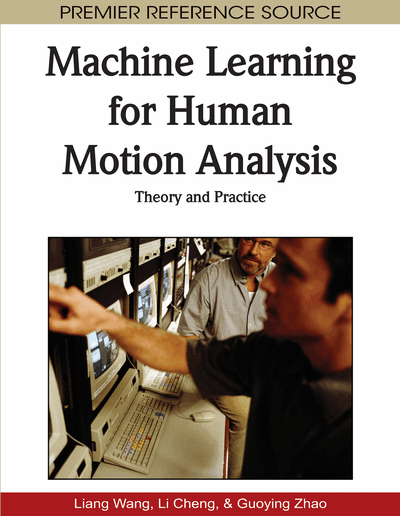# KSM Based Machine Learning for Markerless Motion Capture

Therdsak Tangkuampien (Monash University, Australia) and David Suter (Monash University, Australia)
DOI: 10.4018/978-1-60566-900-7.ch005

## Abstract

A marker-less motion capture system, based on machine learning, is proposed and tested. Pose information is inferred from images captured from multiple (as few as two) synchronized cameras. The central concept of which, we call: Kernel Subspace Mapping (KSM). The images-to-pose learning could be done with large numbers of images of a large variety of people (and with the ground truth poses accurately known). Of course, obtaining the ground-truth poses could be problematic. Here we choose to use synthetic data (both for learning and for, at least some of, testing). The system needs to generalizes well to novel inputs:unseen poses (not in the training database) and unseen actors. For the learning we use a generic and relatively low fidelity computer graphic model and for testing we sometimes use a more accurate model (made to resemble the first author). What makes machine learning viable for human motion capture is that a high percentage of human motion is coordinated. Indeed, it is now relatively well known that there is large redundancy in the set of possible images of a human (these images form som sort of relatively smooth lower dimensional manifold in the huge dimensional space of all possible images) and in the set of pose angles (again, a low dimensional and smooth sub-manifold of the moderately high dimensional space of all possible joint angles). KSM, is based on the KPCA (Kernel PCA) algorithm, which is costly. We show that the Greedy Kernel PCA (GKPCA) algorithm can be used to speed up KSM, with relatively minor modifications. At the core, then, is two KPCA’s (or two GKPCA’s) - one for the learning of pose manifold and one for the learning image manifold. Then we use a modification of Local Linear Embedding (LLE) to bridge between pose and image manifolds.
Chapter Preview
Top

## 1.1 Overview

Humans can look at an image of a human and ``see'' what pose they are in (i.e., can infer, to some accuracy, the joint angles). We have built a system to do something similar: pose information is inferred from images captured from multiple (as few as two) synchronized cameras. The central concept of which, we call: Kernel Subspace Mapping (KSM)(Section 1.5).

The concept can be seen in Figure 1. The image (we extract silhouettes) to pose mapping is to be learnt (left hand side) and then used (right hand side). The learning could be done with large numbers of images of a large variety of people (and with the ground truth poses accurately known). Of course, obtining the ground-truth poses could be problematic: In principle, this could be done using a commercial (expensive) motion capture system but we choose to use synthetic data (both for learning and for, at least some of, testing). Of course, the system needs to generalizes well to novel inputs. That is unseen poses (not in the training database) and unseen actors. For the learning we use a generic and relatively low fidelity computer graphic model (left of the figure) and for testing we sometimes use a more accurate model (made to resemble the first author).

Figure 1.

Diagram to summarize the training and testing process of Kernel Subspace Mapping, which learns the mapping from image to the normalized Relative Joint Centers (RJC) Pose space (section 1.3.1). Note that different mesh models are used in training and testing. The generic model [top left] is used to generate training images, whereas the accurate mesh model of the author (Appendix 3) is used to generate synthetic test images.

What makes machine learning viable for human motion capture is that a high percentage of human motion is coordinated [Safonova et al, 2004; Char and Hodgins, 2005]. Indeed, it is now relatively well known that there is large redundancy in the set of possible images of a human (these images form som sort of relatively smooth lower dimensional manifold in the hug dimensional space of all possible images) and in the set of pose angles (again, a lowdimensional and smooth sub-manifold of the moderately high dimensional space of all possible joint angles). Figure 7 shows these subspaces.

Figure 7.

Diagram to highlight the relationship between human motion de-noising (section 1.2), Kernel Principal Components Analysis (KPCA) and Kernel Subspace Mapping (KSM)

There have been many experiments on the application of learning algorithms (such as Principal Components Analysis (PCA) [Jolliffe, 1986; Smith, 2002] and Locally Linear Embedding (LLE) [Saul and Roweis, 2000]) in learning low dimensional embedding of human motion [Elgammal and Lee, 2004; Bowden, 2000; Safonova et al, 2004]. Similarly, there have been many markerless motion capture techniques based on machine learning [Elgammal and Lee, 2004; Urtasun et al, 2005; Grauman et al, 2003; Agarwal and Triggs, 2006; Ren at al, 2005].

Note: in contrast to [Grauman et al, 2003; Elgammal and Lee, 2004], KSM can estimate pose using training silhouettes generated from a single generic model (Figure 1 [top left]). To ensure the robustness of the technique and test that it generalizes well to previously unseen1 poses from a different actor, a different model is used in testing (Figure 1 [top right]). Results are presented in section 1.5.3, which shows that KSM can refer accurate human pose and direction, without the need for 3D processing (e.g. voxel carving, shape form silhouettes), and that KSM works robustly in poor segmentation environments as well.

## Complete Chapter List

Search this Book:
Reset